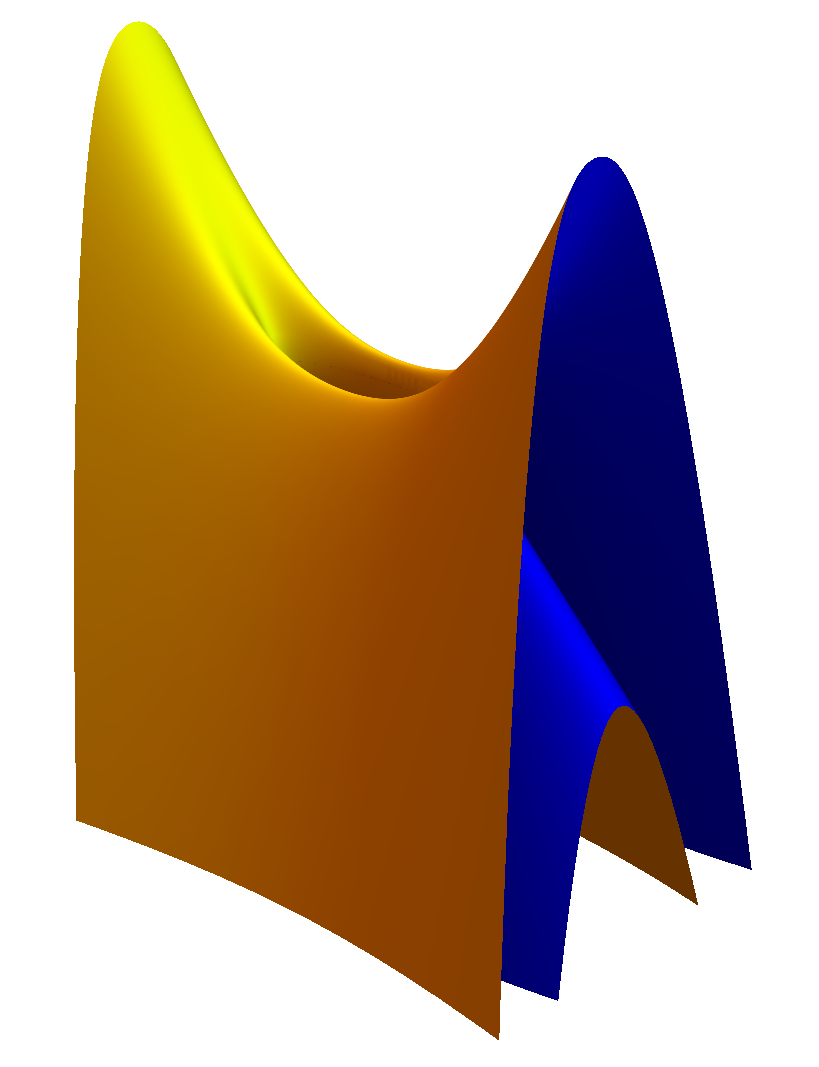# Geometry mini-course: F. Martín (U Granada)Geometry mini-course on self-translating solitons as minimal surfaces.

Abstract: A translator is a surface $M$ in $\mathbb{R}^3$ such that $M+ t v$ is a Mean Curvature Flow, for a unit vector $v$. Translators can be seen (after a rotation), as minimal surfaces in $\mathbb{R}^3$ endowed with the metric $g= e^{-z} (dx^2+dy^2+dz^2)$.
We are going to study a class of translators that plays a role similar to the minimal surfaces with finite total curvature in Euclidean $3$-space. We call them translators of finite type. These translators are characterized by the property that the number of tangencies of $M$ with any foliation by complete translating graphs is bounded by a fixed constant.